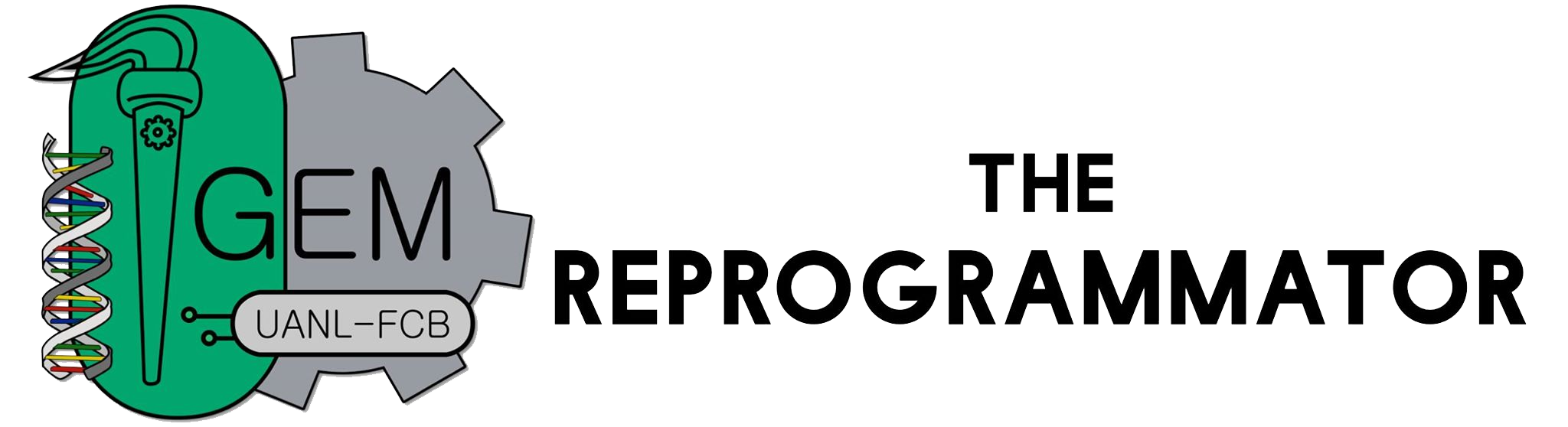# Team:UANL Mty-Mexico/MathModel/Protein

(diff) ← Older revision | Latest revision (diff) | Newer revision → (diff)Math Model
 Protein Production Model
 The transcription of genes begins with the binding of transcription elements onto regulatory sites. The transcription rate is heavily dependant on how strong or weak these sites are. In our project, protein feedback will not be taken into account as there is no regulatory region capable of interacting with the products. We assume that both mRNA and protein synthesis have a constant rate of production, which does not vary throughout the process. Each component degrades randomly and is recycled within the cell. Transcription The average transcription speed in E.coli is 70nt/s. Assuming all the transcription in our circuit works in such speed, we can calculate the maximum transcription rate for each transcription equation by using this formula: Maximum Transcription Rate = Transcription Speed(nt/min)/Gene Length(bp)=4200/Gene Length (nM/min) Translation The average translation speed in E.coli is 40Aa/s. Also assuming all the translation in our circuit works in the same speed, we can calculate the theoretical transcription rate. However, in wetlab, we can use different rbs to regulate the translation process, thus, the translation rate can be written as: Translation Rate = RBS * Translation Speed(Aa/min)/Protein Length(Aa) = 2400RBS/Protein Length (min^-1) This transformation does not change the degree of freedom of our system. However, this does limit the range of parameters since the strength of RBS can not be too extreme. Cell division rate We have assume the period of cell division is 30 mins, which means the "degradation rate" in our model is actually the sum of degradation rate of the substance(1/half life) and cell division rate(1/30 mins). RNA degradation rate From Ref1(Belasco 1993) and Ref2(Genome Biology 2006, 7:R99), we have decided that all the mRNA in our system have a half life of 4.4 mins. \begin{equation} \frac{d[GFP]}{dt}= k_{translation}[mRNA] - k_{degradation} \end{equation} \begin{equation} \frac{d[TALEN]}{dt}= k_{translation}[mRNA] - k_{degradation} \end{equation} These equations would describe the change of concentration of both GFP (our reporter) and the TALEN. As previous teams have described the promoter activity, we expect our system to behave similarly as previously reported.
 Thank you for visiting our wiki. Follow us in: